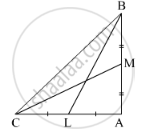Share

# In ∆Abc, ∠Bac = 90°, Seg Bl and Seg Cm Are Medians of ∆Abc. Then Prove That: 4(Bl2 + Cm2) = 5 Bc2 - Geometry

ConceptPythagoras Theorem

#### Question

In ∆ABC, ∠BAC = 90°, seg BL and seg CM are medians of ∆ABC. Then prove that:
4(BL+ CM2) = 5 BC2#### Solution

Given : Δ ABC right angled at A i.e; A = 90°. where BL and CM are the median.

To Prove : 4( BL2 + CM2 ) = 5BC2

Proof :
Since BL is the median,
AL = CL = 1/2 AC                   ...(1)

Similarly, CM is the median
AM = MB = 1/2AB                 ...(2)
We know that, by pythagoras theorem
(Hypotenuse)2 = (Height)2 + (Base)2

In ΔBAC,
(BC)2 = (AB)2 + (AC)2                   ...(4)

In ΔBAL,
(BL)2 = AB2 + AL           ...(From 1)
("BL")^2 = AB^2 + ((AC)/2)^2

("BL")^2 = AB^2 + (AC)^2/4

("BL")^2 = [ 4("AB")^2 + ("AC")^2 ]/4

4"BL"^2 = 4("AB")^2 + "AC"^2         ...(5)

In ΔMAC,
("CM")^2 = ("AM")^2 + ("AC")^2   ...( From 2)

("CM")^2 = (("AB")/2)^2 + ("AC")^2

("CM")^2 = [("AB")^2 + 4("AC")^2 ]/4

4"CM"^2 = "AB"^2 + 4"AC"^2          ...(6)

From 4,5 and 6
(BC)2 = (AB)2 + (AC)2
4"BL"^2 = 4("AB")^2 + "AC"^2
4"CM"^2 = "AB"^2 + 4"AC"^2

4"BL"^2 + 4"CM"^2 = 4"AB"^2 + "AC"^2 + "AB"^2 + 4"AC"^2
4( "BL"^2 + "CM"^2 ) = 5"AB"^2 + 5"AC"^2
4( "BL"^2 + "CM"^2 ) = 5( "AB"^2 + "AC"^2 )
4( "BL"^2 + "CM"^2 ) = 5"BC"^2

Is there an error in this question or solution?

#### APPEARS IN

Balbharati Solution for Balbharati Class 10 Mathematics 2 Geometry (2018 to Current)
Chapter 2: Pythagoras Theorem
Problem Set 2 | Q: 11 | Page no. 45

#### Video TutorialsVIEW ALL 

Solution In ∆Abc, ∠Bac = 90°, Seg Bl and Seg Cm Are Medians of ∆Abc. Then Prove That: 4(Bl2 + Cm2) = 5 Bc2 Concept: Pythagoras Theorem.
S